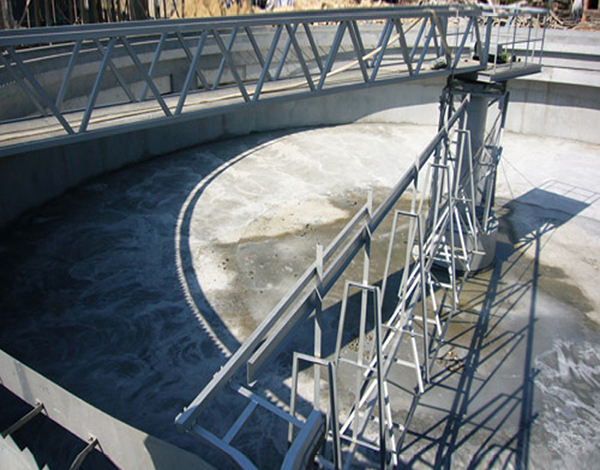# 超纯水处理设备常用单位换算，收藏备用

### 发布日期：2018-08-10 作者： 点击：1L（升）

=0.001 m³（立方米）

=1 dm3 （立方分米）=1L（升）

=1000 cm3（立方厘米）=1mL（毫升）

1kgf(千克力)=9.8N（牛）

1kgf/mm2=9.8Mpa

1 N（牛）=0.22lbf（磅）

1MPa（兆帕）

=10bar（巴）

=103KPa（千帕）

=106Pa（帕）

=145Psi（磅/平方英寸）

1mmHg（1毫米汞柱）= 13.6mmH2O（13.6毫米水柱）= 133.32 Pa（帕）

1mmH2O(毫米水柱)= 9.8Pa（帕）

1 atm（标准大气压）=760mmHg=0.101Mpa

质量单位

1T（吨）

=103Kg（千克）

=10-6g（克）

=10-9μg（微克）

1m（米）

=10dm（分米）

=100cm（厘米）

=1000mm（毫米）

=106μm（微米）

=109nm（纳米）

1inch（英寸）

=12ft (英尺)

=25.4mm（毫米）

1m2（平方米）

=100dm2（平方分米）

=104cm2（平方厘米）

=106mm2（平方毫米）

1inch2（平方英寸）

=12ft2 (平方英尺)

=25.4mm2（平方毫米）

1μs/cm（微西门子/厘米）

=10-4s/m(西门子/米)

=0.1ms/m(毫西门子/米)

10MΩ·cm（兆欧·厘米）=0.1μs/cm（微西门子/厘米）

1mg/L（毫克/升）

=1ppm

=103ppb

=106ppt

==10-3μg/L（微克/升）

1mol/L（摩尔/升）

=1000mmol/L（毫摩尔/升）

1加仑/分(gpm)=0.272立方米/小时(m3/h)=272升/小时(L/h)=4.53L/min

1格令/加仑(grain/gal)=17.1毫克/升(mg/L)

1格令/加仑(grain/gal)=0.342毫克当量/升(mgN/L)

CaCO3的克当量为50，即1克当量CaCO3的质量为50g;

1毫克当量/升=50毫克/升

(1千格令=1.297克当量)

1psi(磅/平方英寸)=0.07kg/cm2=0.006895兆帕(MPa)

1)总交换容量(克当量)=树脂体积(m3)×1000

2)周期总制水量

Q(m3)=总交换容量(mol)÷原水硬度(mmol/L)

Q(m3)=总交换容量(克当量)÷原水硬度(毫克当量/升)

3)周期盐耗(公斤)

=总交换容量(克当量)×(0.08～0.1)公斤/克当量

4)盐阀盐液高度计算